# Insert 6

Insert four harmonic means between 3/7 and 3/19

a2 =  0.355
a3 =  0.303
a4 =  0.2643
a5 =  0.2344

### Step-by-step explanation: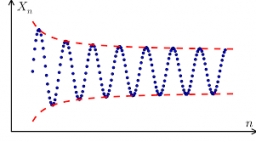Did you find an error or inaccuracy? Feel free to write us. Thank you!Tips to related online calculators
Looking for calculator of harmonic mean?
Looking for a statistical calculator?
Need help to calculate sum, simplify or multiply fractions? Try our fraction calculator.

## Related math problems and questions:

• Insert 7Insert five harmonic means between 3 and 18
• Insert 5Insert five harmonic means between 1/2 and 1/26
• HP - harmonic progressionDetermine the 10th term of the harmonic progression 6,4,3,…
• Harmonic seriesInsert four members between 5/3 and 5/11 to form harmonic series (means).
• Harmonic mean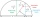If x, y, z form a harmonic progression, then y is the harmonic mean of x and z. Find the harmonic mean of the numbers 6 and 5.
• Harmonic meanHarmonic means of 6 and 12
• Insert 4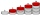Insert three arithmetic means between 3 and 63.
• Insert 3Insert five arithmetic progression members between -7 and 3/2.
• Harmonic 4The harmonic mean of -6 and 5.
• Insert AP memberInsert arithmetic means between 75 and 180.
• HP - harmonic progressionDetermine the 8th term of the harmonic progression 2, 4/3, 1,…
• Sort fractionsWhich is larger 3/7, 3/8, 3/9, 3/6 =
• Mailbox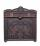Mailbox is opening at regular intervals 3 times a day. The first time is opened at 8:00 and the last at 19:00. Calculate hours when mailbox is opened during day.
• Sequence 11What is the nth term of this sequence 1, 1/2, 1/3, 1/4, 1/5?
• What areWhat are the four terms between 4 and 19?
• TractorsFields go plow two tractors with various performances. The first tractor plows the whole field in 11 hours, the second tractor plows the whole field 19 hours longer. How long take plow the whole field with two tractors?
• Car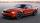The car goes from point A to point B at speed 86 km/h and back 53 km/h. If it goes there and back at speed 67 km/h trip would take 10 minutes shorter. What is the distance between points A and B?